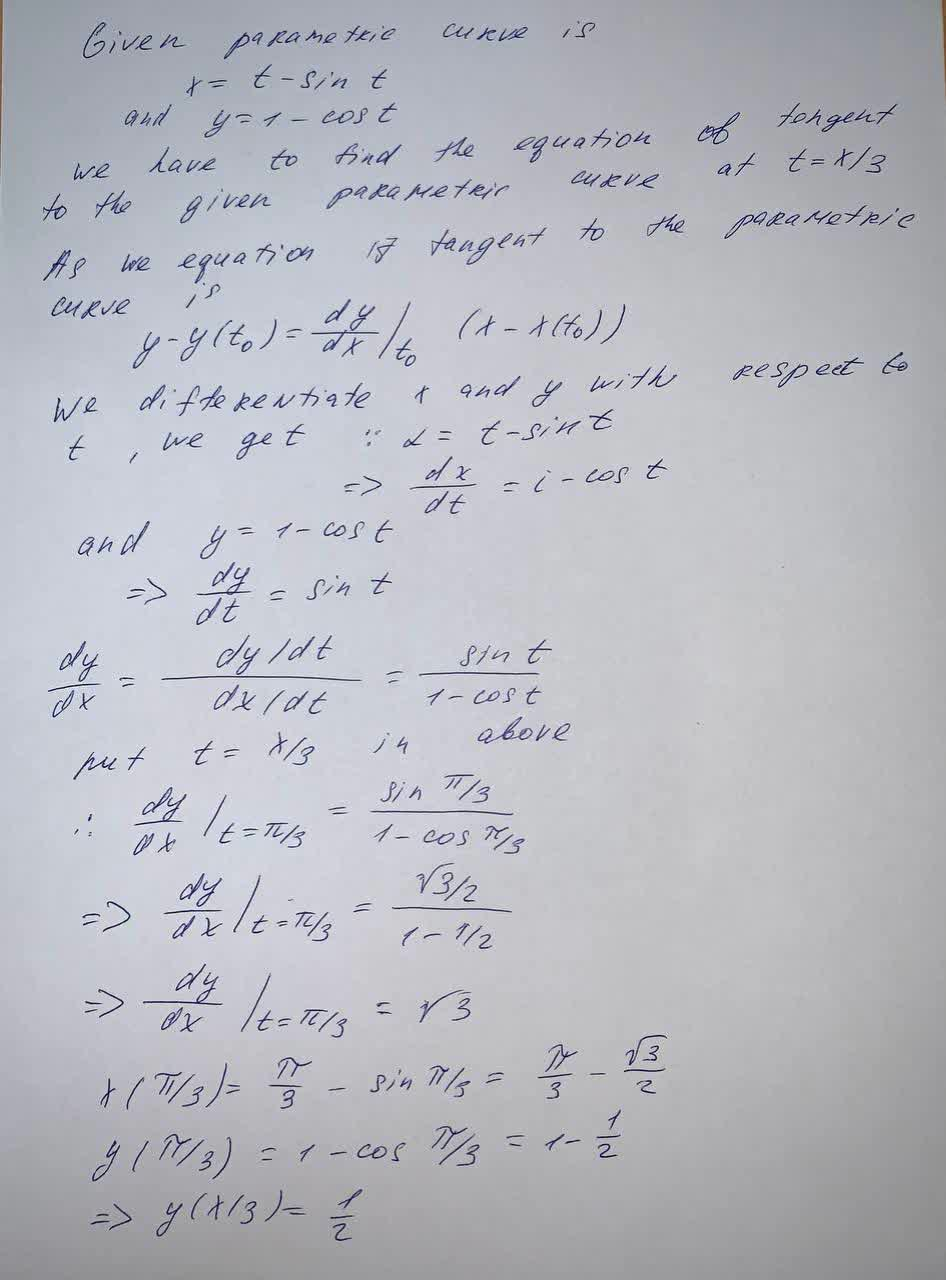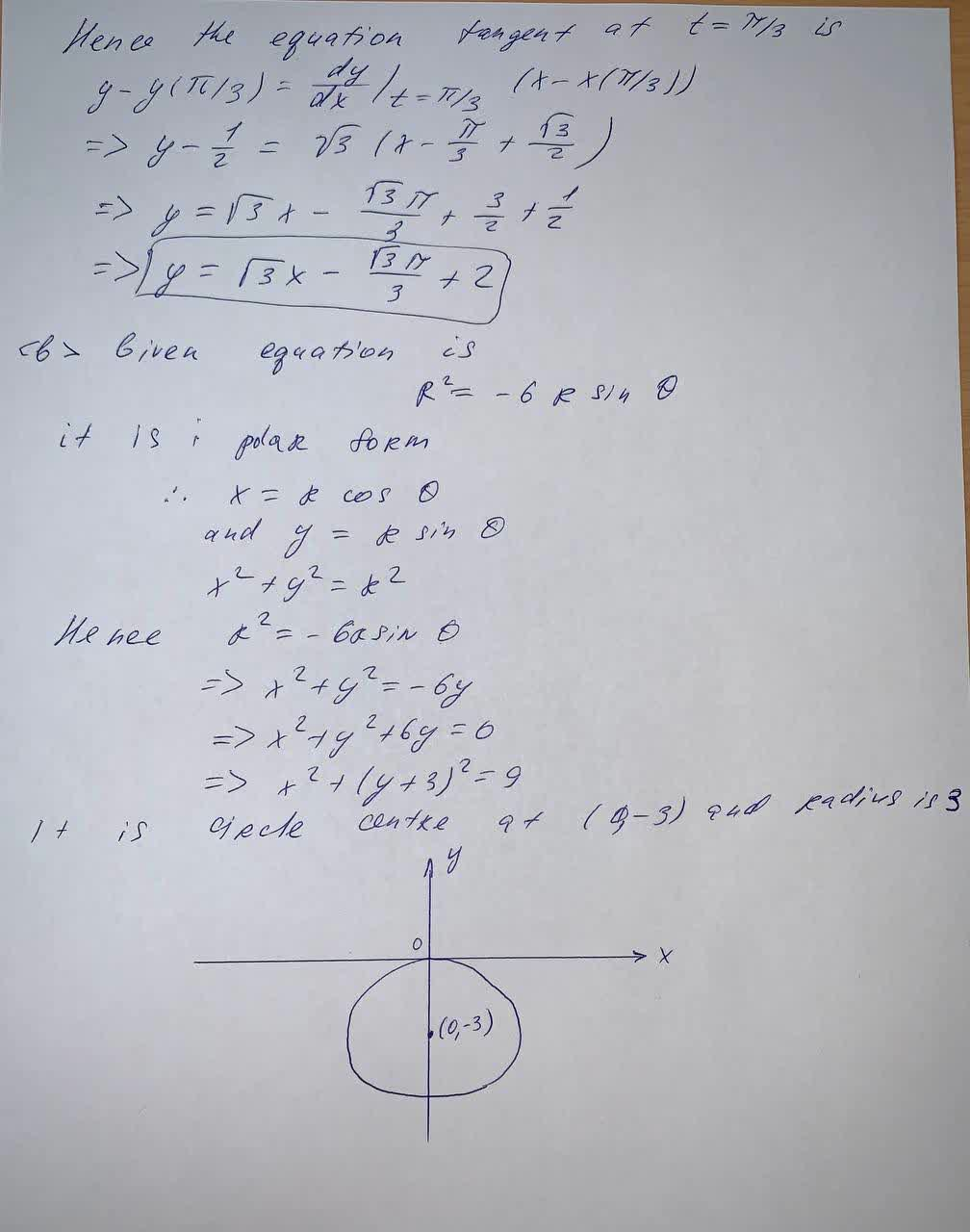x = t− sin t, y = 1 − =cos t curve given by the parametric equationWierzycaz 2021-09-11 Answered

$$x = t− \sin t$$
$$y = 1 − \cos t −$$ curve given by the parametric equation $$\displaystyle=\frac{\pi}{{3}}$$ in point
Find the equation of the tangent line

• Questions are typically answered in as fast as 30 minutes

Solve your problem for the price of one coffee

• Math expert for every subject
• Pay only if we can solve ittabuordy

Given that: